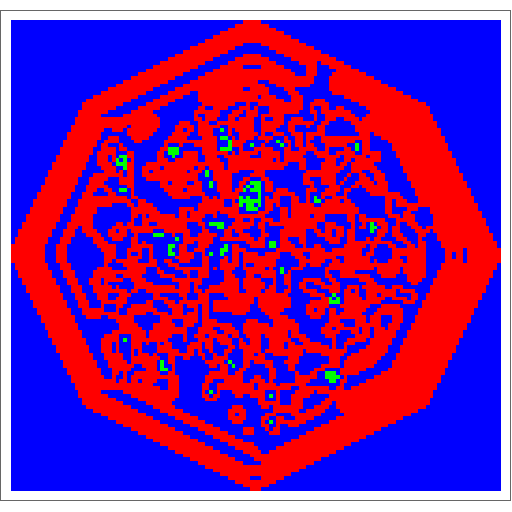#Wolfram Summer School

# Alumni# Ben Abramowitz

Science and Technology

Class of 2016

## Bio

Ben graduated from Tulane University in 2016 with a BA in economics, a BS in mathematics and computer science and a minor in physics. In the fall he will begin pursuing his PhD in computer science at Rensselaer Polytechnic Institute. His current areas of interest are artificial intelligence and algorithmic game theory, and in his spare time he watches an unhealthy number of TED Talks.

## Project: Stable Roommates

Suppose we have a group of students that we would like to split into pairs of two. Our goal is to find the best way to match these students. This problem is commonly known as the stable-roommate problem. In the version of the problem where the exact utilities (cardinal preferences) of the students (agents) are known, there are simple procedures like Irving’s algorithm that produce stable matches, if they exist. However, in most real-life situations, the true values of the agents’ cardinal preferences are unknown. It is more realistic to assume that we only know how the agents rank each other, from most preferred to least preferred (ordinal preferences). For the purpose of demonstration, the model contains 12 agents and the algorithms run over 10,000 instances of the problem.

To evaluate the different ordinal algorithms for producing matches, we take a utilitarian approach by assuming that the agents’ ordinal preferences reflect hidden, underlying cardinal preferences. We can then compare the performance of the matches based on the ordinal preferences to the optimal matches based on the cardinal preferences.

Here we consider two ways in which an agent might form their ordinal preferences. The first way is selfishly (directed), in which an agent only considers their own utility in their preferences. The second way is cooperatively (undirected), in which an agent considers the utility of their potential partner so that each matching provides the same utility for both agents. An agent’s popularity is determined by the sum of their rankings from the other agents. (Directed and undirected refer to the types of graphs that would represent the cardinal preferences, although their ordinal preferences will not be symmetric in either case).

This project would not have been possible without Christopher Wolfram’s instruction, as well as guidance from Jason Cawley, Ian Johnson, Todd Rowland and Stephen Wolfram.

## Favorite 3-Color 2D Totalistic Cellular Automaton

Rule 517386284• 21st Annual Wolfram Summer School
• Bentley University, Waltham, MA, USA
• June 25–July 15, 2023